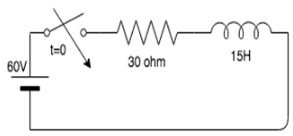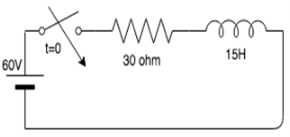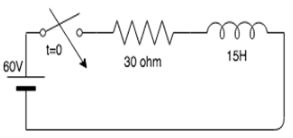Courses

# Test: Transients In LR Networks

## 10 Questions MCQ Test Basic Electrical Technology | Test: Transients In LR Networks

Description
This mock test of Test: Transients In LR Networks for Electrical Engineering (EE) helps you for every Electrical Engineering (EE) entrance exam. This contains 10 Multiple Choice Questions for Electrical Engineering (EE) Test: Transients In LR Networks (mcq) to study with solutions a complete question bank. The solved questions answers in this Test: Transients In LR Networks quiz give you a good mix of easy questions and tough questions. Electrical Engineering (EE) students definitely take this Test: Transients In LR Networks exercise for a better result in the exam. You can find other Test: Transients In LR Networks extra questions, long questions & short questions for Electrical Engineering (EE) on EduRev as well by searching above.
QUESTION: 1

### An RL network is one which consists of ____________

Solution:

An R-L network is a network which consists of a resistor which is connected in series to an inductor.

QUESTION: 2

### At DC, inductor acts as _____________

Solution:

At DC, the inductor acts as short circuit because the inductive resistance is zero. The frequency of a DC circuit is 0. The inductive resistance=(2*pi*f*L). Therefore, if the frequency is 0, the inductive resistance is zero and it acts as an short circuit.

QUESTION: 3

### In an RL series circuit, when the switch is closed and the circuit is complete, what is the response?

Solution:

In an RL series circuit, the response decays with time because according to the equation, there is an exponential decrease in the response.

QUESTION: 4

If the switch is closes at t=0, what is the current in the circuit?Solution:

Initially, when the switch is open, the current in the circuit is 0. As soon as the switch is closes at t=0+, the inductor acts as an open circuit, hence the current in the circuit is zero.

QUESTION: 5

What is the voltage across the inductor at t=0?​Solution:

Initially, when the switch is open, the current in the circuit is 0. As soon as the switch is closes at t=0+, the inductor acts as an open circuit, hence the current in the circuit is zero. Since the current in the circuit is zero, there is no voltage drop across the resistor and the voltage across the inductor is equal to the supply voltage, which is equal to 60V.

QUESTION: 6

What is the expression for current in the given circuit?​Solution:

At t = 0+ the current in the circuit is zero. Therefore at t = 0+, i = 0, 0 = c + 2, c = -2. Substituting the value of c in the current equation, we have i = 2(1-e-2t)A.

QUESTION: 7

At steady state, inductor acts as ____________

Solution:

At steady state, inductor acts as a short circuit because at steady state maximum current flows across the inductor. Hence it acts as a zero resistance source or an open circuit.

QUESTION: 8

At steady state, the current in the inductor is?

Solution:

At steady state maximum current flows in the inductor because it acts as an open circuit.

QUESTION: 9

Initially, when the switch in a series RL circuit is closed, the inductor acts as?

Solution:

Initially, when the switch in a series RL circuit is closed, the inductor acts as an open circuit. This is because the current gradually increases as the time increases. At t=0, the current in the inductor is zero, hence it acts as an open circuit.

QUESTION: 10

Initially, when the switch in a series RL circuit is closed, the current in the inductor is?

Solution:

Initially, when the switch in a series RL circuit is closed, the inductor acts as an open circuit. Current in an open circuit is zero, hence the inductor current is zero.